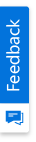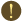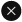We use cookies to give you the best experience on our website. If you continue to browse, then you agree to our privacy policy and cookie policy.Unfortunately, activation email could not send to your email. Please try again.# How to frame the equivalent of TIMESTAMPDIFF() function belonging to MySQL in dashboard designer using expressions?

Platform: Dashboard Platform |
Control: Dashboard Datasources
Tags:

 TIMESTAMPDIFF function is used to return a value after subtracting a date or datetime expression from another expression. Also, it is not necessary that both the expressions are of same type. One may be a date, and another a datetime. A date value is treated as a datetime with a default time part '00:00:00'. The unit for the result is given by another argument. Syncfusion Dashboard Designer doesn’t have the support for TIMESTAMPDIFF function directly. However, we can achieve the same result using other functions in expression editor. Syntax in MySQL: TIMESTAMPDIFF(unit, datetime_expr1,datetime_expr2) The unit should be one of the following: Microseconds, Seconds, Minute, Hour, Day, Week, Month, Quarter, Year. For example, we have taken two date columns named as OrderDate and ShippedDate.Below we have provided steps to get a resultant value after subtracting a date time expression from another based on each unit. STEP 1: Create an expression using first date column "OrderDate".STEP 2: Create an expression using second date column "ShippedDate".STEP 3: Use the below formula to get TIMESTAMPDIFF based on DAY. Difference in Days: DAYDIFF([DateTimeExpr1],[DateTimeEpr2])STEP 4: Use the below formula to get TIMESTAMPDIFF based on Hour. Difference in Hours: [Difference in Days]*24STEP 5: Use the below formula to get TIMESTAMPDIFF based on Minute. Difference in Minutes: [Difference in Hours]*60STEP 6: Use the below formula to get TIMESTAMPDIFF based on Second. Difference in Seconds: [Difference in Minutes]*60STEP 7: Use the below formula to get TIMESTAMPDIFF based on Micro Second. Difference in MicroSeconds: [Difference in Seconds]*POWER(10,6)STEP 8: Use the below formula to get TIMESTAMPDIFF based on Week. Difference in Weeks: ([Difference in Days]-([Difference in Days]%7))/7To calculate difference based on Year, Month and Quarter, you need to create some additional expressions. STEP 9: Use the below formula to find number of year difference. It returns year difference, if DateTimeEpr2 is greater than DateTimeExpr1 else 0. Number of Years: IF(YEAR([DateTimeEpr2])>YEAR([DateTimeExpr1]) AND MONTH([DateTimeEpr2])>=MONTH([DateTimeExpr1]) AND DAY([DateTimeEpr2])>=DAY([DateTimeExpr1]), YEAR([DateTimeEpr2])-YEAR([DateTimeExpr1]), 0)STEP 10: Use the below formula to find number of year difference. If month or day difference of DateTimeEpr2 is less than DateTimeExpr1, it returns -1 to subtract from previous expression result else returns previous expression result. Number of years based on month and day: IF([Number of Years] = 0 AND YEAR([DateTimeEpr2])>YEAR([DateTimeExpr1]) AND (MONTH([DateTimeEpr2]) 0, YEAR([DateTimeEpr2])-YEAR([DateTimeExpr1])-1, [Number of Years])STEP 12: Use below formula to calculate month difference based on year and month of dates. Number of Months :((YEAR([DateTimeEpr2])-YEAR([DateTimeExpr1]))*12)+(MONTH([DateTimeEpr2])-MONTH([DateTimeExpr1]))STEP 13: Use below formula to calculate the month value based on the remaining days since Previous Year.  Remaining days: IF([DateTimeExpr1] > [DateTimeEpr2] AND DAY([DateTimeExpr1]) < DAY([DateTimeEpr2]), -1, 0)STEP 14: Use the below formula, to calculate the Month value based on the upcoming coming days from now. Add Days: IF([DateTimeExpr1] > [DateTimeEpr2] AND DAY([DateTimeExpr1]) < DAY([DateTimeEpr2]), 1, 0)STEP 15: Use the below formula to get TIMESTAMPDIFF based on Month. Difference in Months: [Number of Months]+[Remaining days]+[Add Days]STEP 16: Use the below formula to get TIMESTAMPDIFF based on Quarter. Difference in Quarter: ROUND([Number of Months]/4)You are using an outdated version of Internet Explorer that may not display all features of this and other websites. Upgrade to Internet Explorer 8 or newer for a better experience.